##### Quantum Physics For Dummies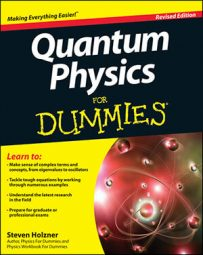Here’s an example that involves finding the rotational energy spectrum of a diatomic molecule. The figure shows the setup: A rotating diatomic molecule is composed of two atoms with masses m1 and m2. The first atom rotates at r = r1, and the second atom rotates at r = r2. What’s the molecule’s rotational energy?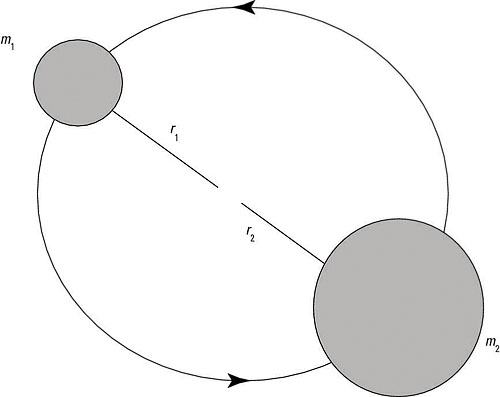A rotating diatomic molecule.

The Hamiltonian is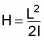I is the rotational moment of inertia, which is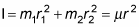where r = |r1r2| and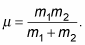Because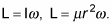Therefore, the Hamiltonian becomes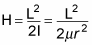So applying the Hamiltonian to the eigenstates, | l, m >, gives you the following: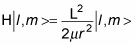And as you know,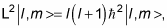so this equation becomes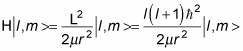And because H | l, m > = E | l, m >, you can see that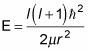And that’s the energy as a function of l, the angular momentum quantum number.